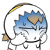# YzmCMS二次开发手册

YzmCMS是采用MVC设计模式开发,基于模块和操作的方式进行访问，采用单一入口模式进行项目部署和访问，无论访问任何一个模块或者功能，只有一个统一的入口。

## 一、基本目录结构

```..../              根目录
..../application   全站应用目录
..../cache         缓存目录[必须可写入]
..../common        全站公共目录[必须可写入]
..../yzmphp        程序核心目录[不建议修改]
..../index.php     程序单一入口文件
..../.htaccess     Apache伪静态文件[如您不是Apache软件，可根据此规则重写伪静态]
..../nginx.conf    Nginx下伪静态文件```

application目录下每一个文件夹都是一个单独的模块（module）

```application
------common（模块公共目录）
------controller（模块控制器目录）
------model（模块模型目录）
------view（模块视图目录）```

## 二、创建一个新控制器

controller目录中的每一个.php文件都是一个控制器，控制器名称都以.class.php后缀结尾。

```<?php

//这两行必须要，后台权限控制
defined('IN_YZMPHP') or exit('Access Denied');
//这两行必须要，后台权限控制

class test extends common {

//访问该控制器的该方法的URL：
public function init() {
echo '程序默认加载控制器中的init方法';
}

//访问该控制器的该方法的URL：
public function mytest() {
echo '这个是mytest方法';
}
}```

URL地址说明：

```//加载其他控制器

//加载系统类

## 三、常见方法说明

D方法

D是data 的首字母，参数为一个表名称，返回的是一个数据表对象（在YzmCMS3.0以下版本中是M方法）

```//实例化一个数据表对象,只传入表名即可，以下所有操作表示在对test表进行操作
\$db = D('test');

//添加内容[成功：返回自动增长的ID，失败：false]
//\$db->insert(数组);
//\$db->insert(array('name'=>'姓名','sex'=>'男'));
//\$db->insert(array('name'=>'姓名','sex'=>'男'), true); //第二个参数选填 如果为真值 则开启实体转义

//删除内容[返回影响行数]
//\$db->delete(array('id>'=>'15'));
//\$db->delete(array(3,4,5), true);  //第二个参数存在时，第一个参数为索引数组，批量删除多个
//\$db->delete(array('1'=>1));   //删除所有数据

//更新内容[返回影响行数]
//\$db->update(array('name'=>'姓名','sex'=>'男123'),array('id'=>'10'));
//\$db->update(array('name'=>'姓名','sex'=>'aaa'),array('id'=>'10'),'1'); //第三个参数选填 如果存在，并为真值 则开启实体转义
//\$db->update('click=click+1',array('id'=>"1"));       //第一个参数不是数组，类似于更新文章点击数的功能

//查询内容 select方式[返回二维数组]

//\$result = \$db->select();
//\$result = \$db->field('uname,id')->select();
//\$result = \$db->where(array('name'=>'%php%'))->select();  //like 查询
//\$result = \$db->where("typeid in (1,2)")->select(); //SQL : select * from user where typeid in (1,2)
//\$result = \$db->where(array('name'=>'%php%'))->limit('0,5')->select(); //like 查询
//\$result = \$db->where(array('name'=>'%php%'))->limit('0,5')->order('id desc')->select(); //like 查询
//\$result = \$db->where(array('sex'=>'男'))->limit('0,5')->select();
//\$result = \$db->field('uname,id')->where(array('sex'=>'男'))->limit('0,3')->order('id desc')->select();
//\$result = \$db->field("sex ,count(sex),avg(height),sum(height) ")->group("sex")->having(" avg(height) >160")->select();
//\$result = \$db->field("sex ,count(sex) as '总个数',avg(height) as '平均身高',sum(height) as '总身高' ")->group('sex')->select();
//\$result = \$db->field("sex ,count(sex) as '总个数',avg(height) as '平均身高',sum(height) as '总身高' ")->group("sex")->having(" 平均身高 >160")->select();

//条件大于写法
//\$res = \$db->where(array('id > '=>21))->select();
//同理，不等于或者小于如下
//\$res = \$db->where(array('id != '=>21))->select();
//P(\$res);

//查询内容 find方式[返回一维数组]
//\$result = \$db->find();
//\$result = \$db->field('uname,id')->find();
//\$result = \$db->where("typeid in (1,2)")->find(); //SQL : select * from user where typeid in (1,2) limit 1
//\$result = \$db->field('id,name,height')->where(array('sex'=>'男'))->find();
//\$result = \$db->field('uname,id')->where(array('sex'=>'男'))->order('id desc')->find();

//查询记录数[返回记录行数]
//\$result = \$db->total();
//\$result = \$db->where(array('age>'=>'12'))->total();

//自定义执行SQL语句 [yzmcms 代表表前缀]
//获取一维数组，一条结果
//获取二维数组

//用于调试程序，输入最后一条SQL语句
//\$db->lastsql();```

YzmCMS 3.8版本新增的数据库操作

```// 新增 one 方法，用来查询某个字段的值 [返回值为字符串类型]

P(\$res);  //运行结果	 string(15) "超级管理员"

\$res = D('article')->field('userid')->where(array('id'=>1))->one();
P(\$res);  //运行结果	 string(1) "1"

//新增 alias 别名方法，用于给表起别名

->limit(5)
->select();

//最后生成的SQL为：

// 注意： join里的 “yzmcms_” 可表示任意的表前缀，无需修改

// 新增 事务处理

\$affair = D('affair');

\$affair->start_transaction();	//开启事务

// 模拟业务流程，执行插入和更新操作

if(\$res && \$res2){
\$affair->commit();  //提交事务
}else{
\$affair->rollback();  //事务回滚
}```

YzmCMS 5.7版本新增的数据库操作

```// insert方法新增第4个参数：replace

// 语法：insert(\$data, \$filter = false, \$primary = true, \$replace = false)
// \$data         要增加的数据，参数为数组
// \$filter       是否开启实体转义
// \$primary 	  是否过滤主键
// \$replace 	  是否为replace
// 返回值：  成功：返回自动增长的ID，失败：false

\$data = array('title'=>'title', 'content'=>'content');
D('test')->insert(\$data, true);

// 新增批量插入方法：insert_all
// 语法：insert_all(\$data, \$filter = false, \$replace = false)
// \$data         要增加的数据，参数为二维数组
// \$filter       是否开启实体转义
// \$replace 	 是否为replace

\$data = array(
array('title'=>'title1', 'content'=>'content1'),
array('title'=>'title2', 'content'=>'content2'),
array('title'=>'title3', 'content'=>'content3'),
);
\$res = D('test')->insert_all(\$data, true);```

M方法

M是model的首字母，参数为一个model类名称，返回的是一个model类对象，意为加载并实例化本模块下的model类

U方法

U是URL的首字母，返回的是一个URL字符串，意为生成URL地址

```// 生成当前模块下的当前控制器的add方法URL地址

U方法可以有第二个参数，即可传参

## 四、二次开发原则

YzmCMS作者开发时比较注重代码简洁、系统高效等特点，所以您在使用YzmCMS做二次开发中也应该遵循以下开发原则：

1.新增功能尽量不要修改系统原文件，建议以模块插件形式开发。

2.用户自定义全局函数写到“common/function/extention.func.php”文件中，不影响系统升级。

3.方法名称和变量名称都以小写字符命名。

4.类文件都以小写字符命名，并以.class.php后缀结尾。

5.所有方法尽可能写上注释等。

###### 随机内容

•网友评论：

不错

2019-07-13 08:46:56 回复•网友评论：

辛苦了，博主2017-04-02 19:51:56 回复